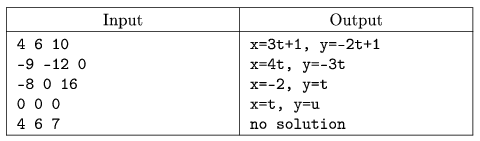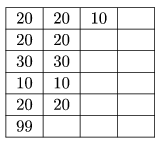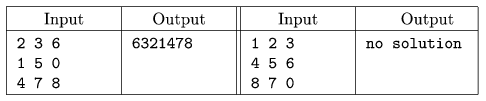Mathematical and Physical Journal
for High Schools
Issued by the MATFUND Foundation
 Already signed up? New to KöMaL?

# KöMaL Problems in Informatics, February 2006

Show/hide problems of signs:## Problems with sign 'I'

Deadline expired on March 16, 2006.

I. 124. Write a program to solve Diophantine equations of the form ax+by=c. The program receives the integers a, b and c having at most four digits in one line from the standard input, separated by spaces. Your program should display the integer solutions in a parametric form in one line as shown below. If there is no solution, print the text ,,no solution''.

Examples:The source code (i124.pas, i124.cpp, ...) is to be submitted.

(10 pont)

solution (in Hungarian), statistics

I. 125. Prepare a LATeX document containing the description of theorems of Ceva and Menelaos. The beginning of the text should contain the usual KöMaL-header (number of the problem, name, city, class, school, e-mail address). You should also create two figures in EPS format to illustrate both theorems. Use the epsfig package to include them into your document. For special characters you may want to use the inputenc package. The LATeX source code (i125.tex) and the EPS figures are to be submitted.

(10 pont)

statistics

I. 126. Prepare an OpenOffice or Excel sheet with the following properties. The user enters at most 100 real numbers into the first column. Your sheet then automatically selects those numbers that are less than the average. These numbers should be listed in the second column without changing their order. Then the program selects numbers from the second column that are less than the average and lists them in the third column. These procedure is repeated until nothing remains.

Example:The sheet (i126.sxc, i126.xls) is to be submitted.

(10 pont)

statistics## Problems with sign 'S'

Deadline expired on March 16, 2006.

S. 15. Write a program that solves Sam Lloyd's famous puzzle of arranging numbered tiles in a 3×3 square. We have 8 tiles in a 3×3 box, so there is one empty position. The tiles are numbered from 1 to 8. In one step one moves an adjacent tile to the empty position. Our aim is to have numbers 1, 2, 3 in the first row, 4, 5, 6 in the second, while 7, 8 and the empty position in the third row.

Your program should read the initial state from the standard input. This will consist of 3 lines, with 3 numbers in each line separated by spaces. A 0 indicates the empty position.

Your program should find the shortest solution (that is, the one consisting of the least number of steps) and print it to the standard output. If there are more than one shortest solutions, any of them can be chosen to be displayed. One step is represented as the number of tile being moved. Numbers should be displayed in one line, without spaces. If there is no solution, simply display ,,no solution''.

Examples:The source code of the program (s15.pas, s15.cpp, ...) should be submitted.

(10 pont)

statistics

\$Var(Body)